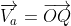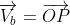# Relative Velocity Between Two Objects: Detailed Analysis

To get an exact idea of the motion of two objects with respect to each other relative velocity is important. So we will talk over the relative velocity between two objects in depth in this article.

Relative velocity is essentially the velocity of one object in relation to another. Consider the following two objects, A and B, which are traveling at different velocities. The velocity of object A in relation to object B, or vice versa, is referred to as relative velocity. It’s also known as the rate of change in the relative position of one object to another over time.

## How to find relative velocity between two objects?

🠊 The technique of determining an object’s velocity requires determining the rate at which the object’s position changes in relation to a stationary surrounding object.

When objects A and B are in relative motion, their respective velocities will also be in relative motion. To acquire object A’s relative velocity with respect to B, one must mathematically impose equal and opposite velocity of B on both object A and B to bring object B to rest.

As a result, the resultant of both velocities (velocity of item A and B) gives us the relative velocity of object A relative to object B.

Relative velocity equations are as follows:

The velocity of an object A in relation to object B can be calculated as follows:

Vab = Va – Vb

The velocity of an object B in relation to object A can be calculated as follows:

Vba = Vb – Va

We can deduce the following from the two expressions:

Vab  = – Vba

However, both magnitudes are equal mathematically and can be represented as:

|Vab |= |Vba|

## What is the relative velocity between two objects when they are moving with same speed in same direction?

🠊 When two objects, A and B, are traveling in the same direction at the same velocity, the angle between them is 0°.

Assume two vehicles A and B are heading in the same direction, i.e., parallel to each other, with the same velocity or speed (since they are moving in the same direction), i.e., Va = Vb.

As a result, the velocity of vehicle A relative to vehicle B is:

Vab = Va – Vb = 0

Similarly, the velocity of vehicle B relative to vehicle A is:

Vba = Vb – Va = 0

It means that if two objects move in the same direction with the same velocity or speed, their relative velocity becomes zero. This demonstrates that another might appear to be at rest for one object.

Plotting a position-time graph for two objects traveling in the same direction at the same speed results in straight parallel lines, as seen in the graph below.

## What is the relative velocity between two objects when they are moving with different speeds in same direction?

🠊 If two vehicles, A and B, are traveling in the same direction at different velocities, there are primarily two scenarios to consider:

(1) Starting points are the same (Va > Vb):

If two vehicles move at different speeds in the same direction with the same starting point and Va > Vb, the person in vehicle B perceives vehicle A to be traveling away from him with velocity:

Vab = Va – Vb

Vehicle B looks to be moving backward to the passenger in vehicle A with velocity:

Vba = Vb – Va = -( Va – Vb)  = -Vab

As a result, both velocities have the same magnitude but opposite signs.

(2) Different starting points:

We may think of two scenarios here:

(i) Assume that vehicle A has a higher velocity than vehicle B, i.e., Va > Vb, and is following vehicle B.

In that situation, vehicle A will eventually overtake vehicle B, as illustrated in their position-time graph.

Vab = Va – Vb ≠ 0

(ii) Consider the situation when Va > Vb and vehicle A is traveling ahead of vehicle B.

In that instance, vehicle B will never be able to overtake vehicle A. Both vehicles’ position-time graphs will not intersect as they move further apart.

Vab = Va – Vb ≠  0

## What will be the relative velocity of two objects when they are moving in opposite directions?

🠊 The angle formed by two objects moving in opposite directions in a straight line is said to be 180°.

Consider two vehicles, A and B, driving in opposing directions in a straight line.

As a result, the velocity of vehicle A in relation to vehicle B is:

Vab = Va -(- Vb) = Va +V

Vehicle B’s velocity in relation to A is similar:

Vba = Vb-(- Va) = Va +V

As a result, we can write:

Vab = Vba

It indicates that if two objects are going in opposite directions in a straight line, each object appears to be moving very quickly compared to the other.

## What is the relative velocity when two objects are moving at an angle?

🠊 Consider the instance of relative velocity, which occurs when two objects, A and B, move at an angle with velocities Va and Vb.&The diagonal will give us the relative velocity if we build a parallelogram, as indicated in the figure. As a result, the magnitude of the parallelogram’s diagonal vector or the relative velocity using the Law of Cosines is:

But, Cos(180°-𝛳) = -Cos𝛳

When two objects travel at an angle, the above equation gives us their relative velocity. We may also derive the same direction case and the opposite direction case from this equation by changing the angle value to 0° and 180°, respectively.

However, as illustrated in the image, if the relative velocity vector Vab forms an angle ꞵ with the velocity of object A, then

But, Sin(180°-𝛳) = Sin𝛳

Or,

## Importance of Relative Velocity:

The importance of relative velocity is summarized below:

• To calculate the velocity of stars and asteroids with respect to earth.
• To measure the distance between any two objects in space.
• To launch a rocket.
• To detect the speed of any object.
• It helps us when an object is moving through the fluid.

## 1. A car traveling on the highway at a velocity of 110 km/h passes a bus traveling at 85 km/h. What is the car’s velocity as seen from the perspective of a bus passenger?

Given:

Velocity of a car Vc = 110 km/h

Velocity of a bus Vb = 85 km/h

To Find:

Relative velocity of car with respect to bus Vcb = ?

Solution:

Since the car and the bus are traveling in the same direction, the car’s relative velocity from the perspective of a bus passenger is:

Vcb = Vc – Vb = (110 -85)km/h = 25 km/h

Thus, the car’s velocity, as seen from the perspective of a bus passenger, is 25 km/h.

## 2. Two cars, some distance apart, begin moving towards each other at speeds of 150 m/s and 200 m/s over a straight road. What is the rate at which they approach each other?

Given:

Velocity of a car 1 V1 = 150 m/s

Velocity of a car 2 V2 = 200 m/s

To Find:

Relative velocity of car 1 with respect to car 2 V12 = ?

Relative velocity of car 2 with respect to car 1 V21 = ?

Solution:

Since both the cars are traveling in the opposite direction, relative velocity:

V12 = V1 + V2 = (150 + 200)m/s = 350 m/s

Similarly,

V21 = V1 + V2 = (150 + 200)m/s = 350 m/s

As a result, the two cars are heading toward each other at a relative velocity of 350 m/s.

#### Summary:

• The velocity of one object in relation to another object is simply called the relative velocity of those two objects.
• Consider two things that are both traveling in the same direction. In that situation, the magnitude of one object’s relative velocity with regard to another will be the difference in the magnitude of their velocities.
• If two objects are moving in the same direction and velocities, their relative velocity will be zero.
• Suppose any two objects are moving in the opposite direction. In that case, the magnitude of the relative velocity of one object with respect to another one will turn out to be the sum of the magnitude of their velocities.

Alpa P. Rajai

I am Alpa Rajai, Completed my Masters in science with specialization in Physics. I am very enthusiastic about Writing about my understanding towards Advanced science. I assure that my words and methods will help readers to understand their doubts and clear what they are looking for. Apart from Physics, I am a trained Kathak Dancer and also I write my feeling in the form of poetry sometimes. I keep on updating myself in Physics and whatever I understand I simplify the same and keep it straight to the point so that it deliver clearly to the readers. You can also reach me at : https://www.linkedin.com/in/alpa-rajai-858077202/